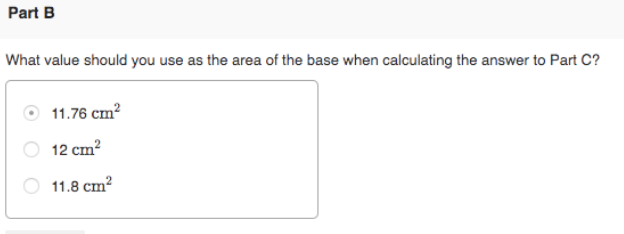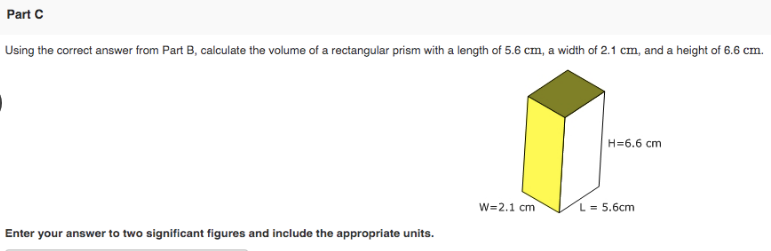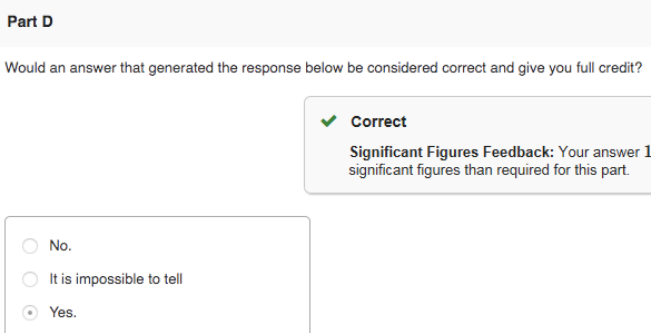# Part B. What value should you use as the area of the base when calculating the answer to Part C? Part C. Using the correct answer from part B, calculate the volume of a rectangular prism with a length of 5.6 cm, a width of 2.1 cm, and a height of 6.6 cm. Enter your answer to two significant figures and include the appropriate units. Part D. Would an answer that generated the response below be considered correct and give you full credit?